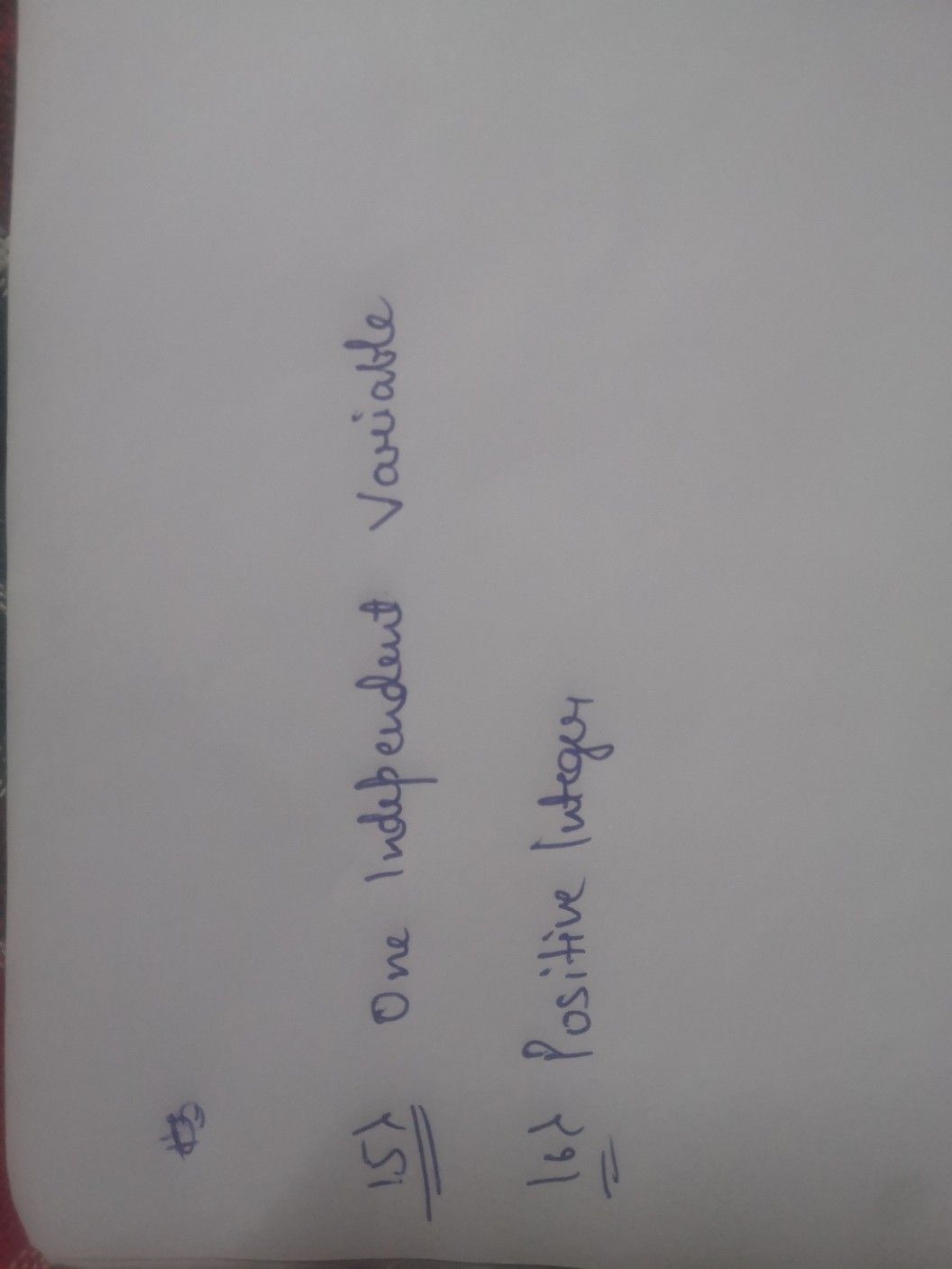Symbol
ProblemWhich of the following is $3n$ exact.14 * ?ordinary differential equation (abäi $2\right)$ $y^{2}dx+\left(2y-2x\right)dx=0$ $O$ $2xdx+\left(x^{2}+3\right)dy=00$ $\left(y+x\right)dx-dx=00$ 0 0 $3dx-3xdx=0$ $A$ differential equation is considered $10.15$ $:1$ be ordinary if it has (abāi $1\right)$ more than one dependent variable O more than one independent variable O one dependent variable one independent variable $16$ * The order of differential equation is always.16 (äbāi $21$ $-$ None of these Rational Number O Positive integer O Real number Negative integer O
1st-6th grade
Calculus
SolutionQanda teacher - Muskan